Level 14 Level 16
Level 15

## Ignore words

Check the boxes below to ignore/unignore words, then click save at the bottom. Ignored words will never appear in any learning session.

Ignore?
Chebyshev's inequality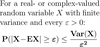Markov's inequalityConvergent in probability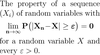Weak law of large numbersAlmost surely convergentStrong law of large numbersFinite variance (as LLN assumption)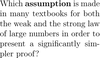Almost sure convergence (vs in probability)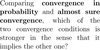Stirling's approximation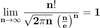CDF of the standard normal distribution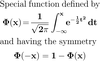Standardizing (or normalizing)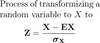De Moivre–Laplace theoremPoisson limit theoremCentral limit theorem (CLT)